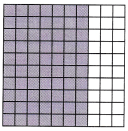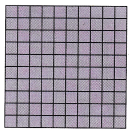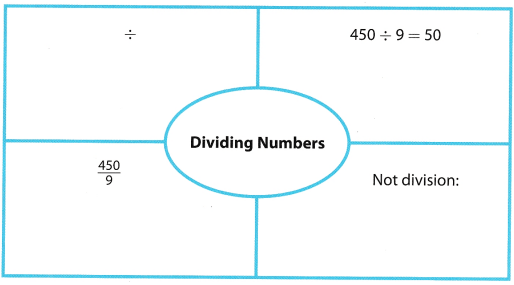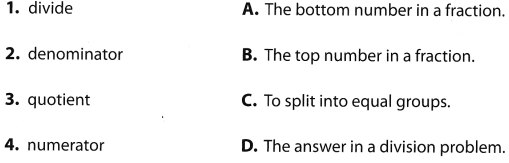# Texas Go Math Grade 6 Module 4 Answer Key Multiplying and Dividing Decimals

Refer to our Texas Go Math Grade 6 Answer Key Pdf to score good marks in the exams. Test yourself by practicing the problems from Texas Go Math Grade 6 Module 4 Answer Key Multiplying and Dividing Decimals.

## Texas Go Math Grade 6 Module 4 Answer Key Multiplying and Dividing Decimals

Write the decimals represented by the shaded square.

Question 1.We have that 10 squares are 10 of 100 equal parts, or $$\frac{10}{100}$$ or 0.1.
Here we have 70 shaded squares, so, they represent 7 × 0.1, or 0.7.

Question 2.We have that 10 squares are 10 of 100 equal parts, or $$\frac{10}{100}$$ or 0.1.
Here we have 70 shaded squares, so, they represent 4 × 0.1, or 0.4.

Question 3.We have that 1 square is 1 of 100 equal parts, or $$\frac{1}{100}$$, or 0.01.
Also, 10 squares are 10 of 100 equal parts, or $$\frac{10}{100}$$, or 0.1.
Here we have 53 shaded squares, we can rewrite them as 503.
So, 3 squares represent 3 × 0.01, or 0.03 and 50 squares represent 50 × 0.1, or 0.5.
Finally, 53 squares represent 0.03 + 0.5 = 0.53

Question 4.Here, we have 100 shaded squares, they are 100 of 100 equal parts, or $$\frac{100}{100}$$, or 1.

Find the product.

Question 5.
0.49 × 10 ___________
Number of zeros in 10 is 1, so, we have to move the decimal point 1 place to the right and we get that the result is 4.9.

Question 6.
25.34 × 1,000 ___________
Number of zeros in 1000 is 3, so, we have to move the decimal point 3 places to the right and we get that the result is 25, 340
Here, because we had two decimal places, we added 0 at the third place because we moved decimal point 3 places.

Question 7.
87 × 100 ___________
Here, the number of zeros in 100 is 2. In this case we will add two zeros to 87 and get that the result is 8,700

Write a numerical expression for the word expression.

Question 8.
20 decreased by 8 _______________
Decreased means “to subtract 8 from 20”, so, required numerical expression would be 20 – 8.

Question 9.
the quotient of 14 and 7 _______
The quotient means the answer in division problem, so, required numerical expression would be $$\frac{14}{7}$$

Question 10.
the difference between 72 and 16 _________
The difference between two numbers means to subtract them, so, required expression would be 72 – 16.

Question 11.
the sum of 19 and 3 __________
The sum of two numbers means to add them, so, required expression would be 19 + 3.

Visualize Vocabulary

Use the ✓ words to complete the chart. You may put more than one word in each section.Understand Vocabulary

Match the term on the left to the definition on the right.